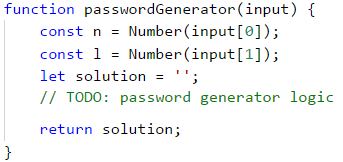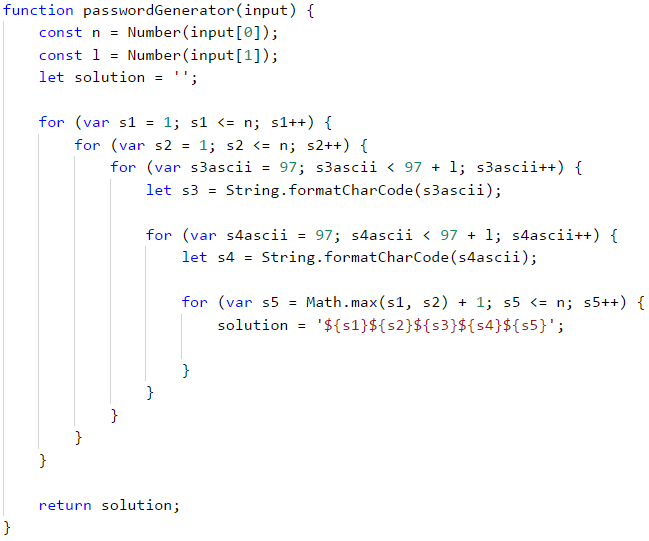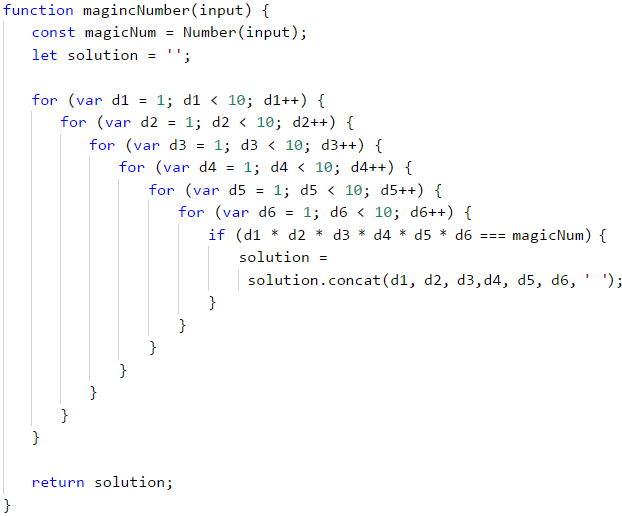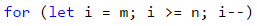# Chapter 7.2. More Complex Loops – Exam Problems

We already learned how to execute a block of commands more than once using a for loop. In the previous chapter, we reviewed some loop structures that would help us solve more complex problems like:

• loops with a step
• nested loops
• while loops
• do-while loops
• infinite loops and breaking out of the loop (break operator)
• try-catch construction

## Exam Problems

Let's start work on solving the following practical exam problems.

Write a program that enters two integers n and l and generates in alphabetical order all possible "dumb" passwords that consist of the following 5 characters:

• Character 1: digit from 1 to n.
• Character 2: digit from 1 to n.
• Character 3: small letter among the first l letters of the Latin alphabet.
• Character 4: small letter among the first l letters of the Latin alphabet.
• Character 5: digit from 1 to n, bigger than first 2 digits.

### Input Data

The input is an array with two integers (arguments): n and l within the range [1 … 9].

### Output Data

Print on the console all "dumb" passwords in alphabetical order, separated by space.

### Sample Input and Output

Input Output Input Output
[ '2', '4' ] 11aa2 11ab2 11ac2 11ad2 11ba2 11bb2 11bc2 11bd2 11ca2 11cb2 11cc2 11cd2 11da2 11db2 11dc2 11dd2 [ '3' , '1' ] 11aa2 11aa3 12aa3 21aa3 22aa3
Input Output Input Output
[ '3' , '2' ] 11aa2 11aa3 11ab2 11ab3 11ba2 11ba3 11bb2 11bb3 12aa3 12ab3 12ba3 12bb3 21aa3 21ab3 21ba3 21bb3 22aa3 22ab3 22ba3 22bb3 [ '4' , '2' ] 11aa2 11aa3 11aa4 11ab2 11ab3 11ab4 11ba2 11ba3 11ba4 11bb2 11bb3 11bb4 12aa3 12aa4 12ab3 12ab4 12ba3 12ba4 12bb3 12bb4 13aa4 13ab4 13ba4 13bb4 21aa3 21aa4 21ab3 21ab4 21ba3 21ba4 21bb3 21bb4 22aa3 22aa4 22ab3 22ab4 22ba3 22ba4 22bb3 22bb4 23aa4 23ab4 23ba4 23bb4 31aa4 31ab4 31ba4 31bb4 32aa4 32ab4 32ba4 32bb4 33aa4 33ab4 33ba4 33bb4

### Hints and Guidelines

We can split the solution of the problem into 3 parts:

• Reading the input – in the current problem, this includes reading two numbers n and l and converting them to numbers.
• Processing the input data – using nested loops to iterate through every possible symbol for each of the five password symbols.
• Printing the output – printing every "dumb" password that meets the requirements.

For reading of input data we will declare two constants const: n and l and also we will declare a variable solution in which we will store a string with the result.#### Printing Output

One of the ways to find a solution for this problem is to create five for nested loops, one for each variable. To ensure that the last digit is greater than the first two, we will use the built-in method Math.max(…):## Problem: Magic Numbers

Write a program that enters a single integer magic number and produces all possible 6-digit numbers for which the product of their digits is equal to the magical number.

Example: "Magic number" → 2

• 111112 → 1 * 1 * 1 * 1 * 1 * 2 = 2
• 111121 → 1 * 1 * 1 * 1 * 2 * 1 = 2
• 111211 → 1 * 1 * 1 * 2 * 1 * 1 = 2
• 112111 → 1 * 1 * 2 * 1 * 1 * 1 = 2
• 121111 → 1 * 2 * 1 * 1 * 1 * 1 = 2
• 211111 → 2 * 1 * 1 * 1 * 1 * 1 = 2

### Input Data

The program reads one integer (argument) within the range [1 … 600 000].

### Output Data

Print on the console all magic numbers, separated by space.

### Sample Input and Output

Input Output Input Output Input Output
2 111112 111121 111211 112111 121111 211111 8 111118 111124 111142 111181 111214 111222 111241 111412 111421 111811 112114 112122 112141 112212 112221 112411 114112 114121 114211 118111 121114 121122 121141 121212 121221 121411 122112 122121 122211 124111 141112 141121 141211 142111 181111 211114 211122 211141 211212 211221 211411 212112 212121 212211 214111 221112 221121 221211 222111 241111 411112 411121 411211 412111 421111 811111 531441 999999

### Hints and Guidelines

The solution of this problem follows the same conception (we have to generate all n combinations of the element). Follow the steps and try to solve the problem on your own:

• Initialize the variable where you will store the magic number.
• Nest six for loops, one for each digit.
• In the last loop, using an if statement, check if the product of the six digits is equal to the magic number.In the previous chapter, we reviewed other loop constructions. Let's look at the sample solution of the same problem using the while loop. First, we need to store the input magical number in a suitable variable. Then we will initialize 6 variables – one for each of the six digits of the searched numbers.Then we will start writing while loops.

• We will initialize first digit: d1 = 1.
• We will set a condition for each loop: the digit will be less than or equal to 10.
• At the beginning of each loop, we set a value of the next digit, in this case: d2 = 1. In the nested for loops, we initialize the variables in the inner loops at each increment of the outer ones. We want to do the same here.
• At the end of each loop, we will increase the digit by one: d++.
• In the innermost loop, we will make the check and if necessary, we add the result into the variable, that stores the solution.As we can see, we can solve a problem using different types of loops. Of course, each task has its most appropriate choice. To practice each type of loop – try to solve each of the following problems with all the learned loops.

## Problem: Stop Number

Write a program that prints on the console all numbers from N to M, that are divisible by 2 and 3 without remainder, in reversed order. We will read one more "stop" number from the console – S. If any of the numbers divisible by 2 and 3 is equal to the stop number, it should not be printed, and the program should end. Otherwise print all numbers up to N, that meet the condition.

### Input Data

Read from the console 3 numbers, each on a single line:

• N – integer number: 0 ≤ N < M.
• M – integer number: N < M ≤ 10000.
• S – integer number: N ≤ S ≤ M.

### Output Data

Print on the console on a single line all numbers, that meet the condition, separated by space.

### Sample Input and Output

1
30
15
30 24 18 12 6 Numbers from 30 to 1, that are divisible at the same time by 2 and 3 without remainder are: 30, 24, 18, 12 and 6. The number 15 is not equal to any, so the sequence continues.
1
36
12
36 30 24 18 Numbers from 36 to 1, that are divisible at the same time by 2 and 3 without remainder are: 36, 30, 24, 18, 12 and 6. The number 12 is equal to the stop number, so we stop by 18.
Input Output
20
1000
36
996 990 984 978 972 966 960 954 948 942 936 930 924 918 912 906 900 894 888 882 876 870 864 858 852 846 840 834 828 822 816 810 804 798 792 786 780 774 768 762 756 750 744 738 732 726 720 714 708 702 696 690 684 678 672 666 660 654 648 642 636 630 624 618 612 606 600 594 588 582 576 570 564 558 552 546 540 534 528 522 516 510 504 498 492 486 480 474 468 462 456 450 444 438 432 426 420 414 408 402 396 390 384 378 372 366 360 354 348 342 336 330 324 318 312 306 300 294 288 282 276 270 264 258 252 246 240 234 228 222 216 210 204 198 192 186 180 174 168 162 156 150 144 138 132 126 120 114 108 102 96 90 84 78 72 66 60 54 48 42

### Hints and Guidelines

The problem can be divided into four logical parts:

• Checking all numbers in the given range, and then running a loop.
• Checking the conditions of the problem according to every number in the given range.
• Printing the numbers.

The First part is ordinary – we read three integer numbers from the console.

We have already seen examples of the second part – initialization of the for loop. It is a bit tricky – the explanation mentions that the numbers have to be printed in reversed order. This means that the initial value of the variable i will be bigger, and from the examples, we can see that it is M. Thus, the final value of i should be N. The fact that we will print the results in reversed order and the values of i, suggests that the step would be decreased by 1.After we have initialized the for loop, it is time for the third part of the problem – checking the condition if the given number is divisible both by 2 and 3 without remainder. We will do this using one simple if condition that we will leave to the reader to do by themselves.

Another tricky part of this problem is that apart from the above check we need to do another one – whether the number is equal to the "stop" number entered from the console on the third line. To do this check, the previous one has to be passed. For this reason, we will add another if statement that we will nest in the previous one. If the condition is true, we need to stop the program from printing. We can do this using a break operator, and it will lead us out of the for loop.

If the condition that checks whether the number is equal with the "stop" number returns a false result, our program should continue to print. This covers the fourth and last part of our program.

## Problem: Special Numbers

Write a program that reads one integer number N and generates all possible special numbers from 1111 to 9999. To be considered special, a number must correspond to the following condition:

• N to be divisible by each of its digits without remainder.

Example: upon N = 16, 2418 is a special number:

• 16 / 2 = 8 without remainder
• 16 / 4 = 4 without remainder
• 16 / 1 = 16 without remainder
• 16 / 8 = 2 without remainder

### Input Data

The input is read from the program and consists of one integer (argument) within the range [1 … 600 000].

### Output Data

Print on the console all special numbers, separated by space.

### Sample Input and Output

3 1111 1113 1131 1133 1311 1313 1331 1333 3111 3113 3131 3133 3311 3313 3331 3333 3 / 1 = 3 without remainder
3 / 3 = 1 without remainder
3 / 3 = 1 without remainder
3 / 3 = 1 without remainder
Input Output Input Output
11 1111 16 1111 1112 1114 1118 1121 1122 1124 1128 1141 1142 1144 1148 1181 1182 1184 1188 1211 1212 1214 1218 1221 1222 1224 1228 1241 1242 1244 1248 1281 1282 1284 1288 1411 1412 1414 1418 1421 1422 1424 1428 1441 1442 1444 1448 1481 1482 1484 1488 1811 1812 1814 1818 1821 1822 1824 1828 1841 1842 1844 1848 1881 1882 1884 1888 2111 2112 2114 2118 2121 2122 2124 2128 2141 2142 2144 2148 2181 2182 2184 2188 2211 2212 2214 2218 2221 2222 2224 2228 2241 2242 2244 2248 2281 2282 2284 2288 2411 2412 2414 2418 2421 2422 2424 2428 2441 2442 2444 2448 2481 2482 2484 2488 2811 2812 2814 2818 2821 2822 2824 2828 2841 2842 2844 2848 2881 2882 2884 2888 4111 4112 4114 4118 4121 4122 4124 4128 4141 4142 4144 4148 4181 4182 4184 4188 4211 4212 4214 4218 4221 4222 4224 4228 4241 4242 4244 4248 4281 4282 4284 4288 4411 4412 4414 4418 4421 4422 4424 4428 4441 4442 4444 4448 4481 4482 4484 4488 4811 4812 4814 4818 4821 4822 4824 4828 4841 4842 4844 4848 4881 4882 4884 4888 8111 8112 8114 8118 8121 8122 8124 8128 8141 8142 8144 8148 8181 8182 8184 8188 8211 8212 8214 8218 8221 8222 8224 8228 8241 8242 8244 8248 8281 8282 8284 8288 8411 8412 8414 8418 8421 8422 8424 8428 8441 8442 8444 8448 8481 8482 8484 8488 8811 8812 8814 8818 8821 8822 8824 8828 8841 8842 8844 8848 8881 8882 8884 8888

### Hints and Guidelines

Solve the problem by yourself using what you learned from the previous two problems. Keep in mind the difference between operators for integer division ( / ) and division with remainder ( % ) in JavaScript.

## Problem: Digits

Write a program that reads from the console 1 integer within the range [100 … 999], and then prints it a predefined number of times – modifying it before each print, as follows:

• If the number is divisible by 5 without remainder, subtract from it its first digit.
• If the number is divisible by 3 without remainder, subtract from it its second digit
• If none of the above-mentioned conditions is valid, add to it its third digit.

Print on the console N lines, and each line has M numbers, that are the result of the above actions.

• N = sum of the first and second digits of the number.
• M = sum of the first and third digits of the number.

### Input Data

The input is read from the program and is an integer (argument) within the range [100 … 999].

### Output Data

Print on the console all integer numbers, that is the result of the above-mentioned calculations in the respective number of rows and columns as in the examples.

### Sample Input and Output

132 129 126 123
120 119 121
123 120 119
121 123 120
(1 + 3) = 4 and (1 + 2) = 3 → 4 lines with 3 numbers in each
Input number 132
132 → division by 3 → 132 - 3 =
= 129 → division by 3 → 129 - 3 =
= 126 → division by 3 → 126 - 3 =
= 123 → division by 3 → 123 - 3 =
= 120 → division by 5 → 120 - 1 =
..... 121 → neither by 5, nor 3 → 121 + 2 = 123
376 382 388 394 400 397 403 409 415 412
418 424 430 427 433 439 445 442 448
454 460 457 463 469 475 472 478 484
490 487 493 499 505 502 508 514 520
517 523 529 535 532 538 544 550 547
553 559 565 562 568 574 580 577 583
589 595 592 598 604 610 607 613 619
625 622 628 634 640 637 643 649 655
652 658 664 670 667 673 679 685 682
688 694 700 697 703 709 715 712 718
10 lines with 9 numbers in each
Input number 376 → neither 5, nor 3 → 376 + 6 → =
= 382 → neither 5, nor 3 → 382 + 6 =
= 388 + 6 = 394 + 6 =
400 → division by 5 → 400 - 3 = 397

### Hints and Guidelines

Solve the problem by yourself, using what you learned from the previous ones. Remember that you will need to define different variables for each digit of the input number.